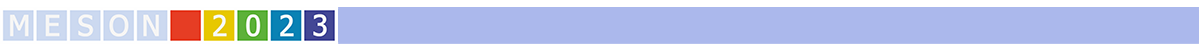#MESON2023

Jun 22 – 27, 2023
Auditorium Maximum
Europe/Warsaw timezone

## Theoretical status of antikaon-nucleon interactions

Jun 26, 2023, 9:00 AM
30m
Medium lecture hall (A and B) (Auditorium Maximum)

Invited

### Speaker

Tetsuo Hyodo (Tokyo Metropolitan University)

### Description

In this talk, we discuss the recent theoretical studies of the strong interaction between an antikaon ($\bar{K}$) and a nucleon ($N$) [1,2]. The isospin $I=0$ channel is of particular interest, as it exhibits an attractive interaction that can generate a quasi-bound state known as the $\Lambda(1405)$ resonance, located below the $\bar{K}N$ threshold. This suggests that the $\bar{K}N$ interaction may also produce quasi-bound states of kaonic nuclei . Furthermore, the quasi-bound picture of the $\Lambda(1405)$ is related to the discussion of hadronic molecules in hadron spectroscopy.

Theoretical description of the $\Lambda(1405)$ in the coupled-channel meson-baryon scattering is developed with chiral SU(3) dynamics . Based on the next-to-leading order (NLO) chiral SU(3) dynamics combined with the precise measurement of kaonic hydrogen by SIDDHARTA, it is shown that there are two resonances between the $\pi\Sigma$ and $\bar{K}N$ thresholds, $\Lambda(1405)$ and $\Lambda(1380)$. We review the current status of the theoretical studies of chiral SU(3) dynamics, including the recently performed NNLO analysis .

Femtoscopic study of the two-particle momentum correlation functions in high-energy collisions has become a new method to extract the hadron-hadron interactions. We present the study on the two-particle correlation function of a $K^-p$ pair in high-energy collisions within the $\bar{K}N$-$\pi\Sigma$-$\pi\Lambda$ coupled-channels framework, which accurately account for all relevant effects, including the Coulomb potential and the threshold energy difference between $K^-p$ and $\bar{K}^0 n$ . Realistic $\bar{K}N$-$\pi\Sigma$-$\pi\Lambda$ potential based on NLO chiral SU(3) dynamics is used. We discuss the resulting $K^-p$ correlation functions in comparison with the recent measurements by the ALICE collaboration under various collision conditions .

 T. Hyodo, W. Weise, arXiv:2202.06181 [nucl-th]
 U.G. Meißner, Symmetry 12, 981 (2020); M. Mai, Eur. Phys. J. ST 230 6, 1593 (2021); T. Hyodo, M Niiyama, Prog. Part. Nucl. Phys. 120, 103868 (2021).
 J.-X. Lu, L.S. Geng, M. Doering, M. Mai, Phys. Rev. Lett. 130, 071902 (2023).
 Y. Kamiya, T. Hyodo, K. Morita, A. Ohnishi and W. Weise, Phys. Rev. Lett. 124, 132501 (2020).
 S. Acharya et al. (ALICE collaboration), Phys. Rev. Lett. 124, 092301 (2020); S. Acharya et al. (ALICE collaboration), Phys. Lett. B 822, 136708 (2021); ALICE collaboration, arXiv:2205.15176 [nucl-ex]

### Primary author

Tetsuo Hyodo (Tokyo Metropolitan University)